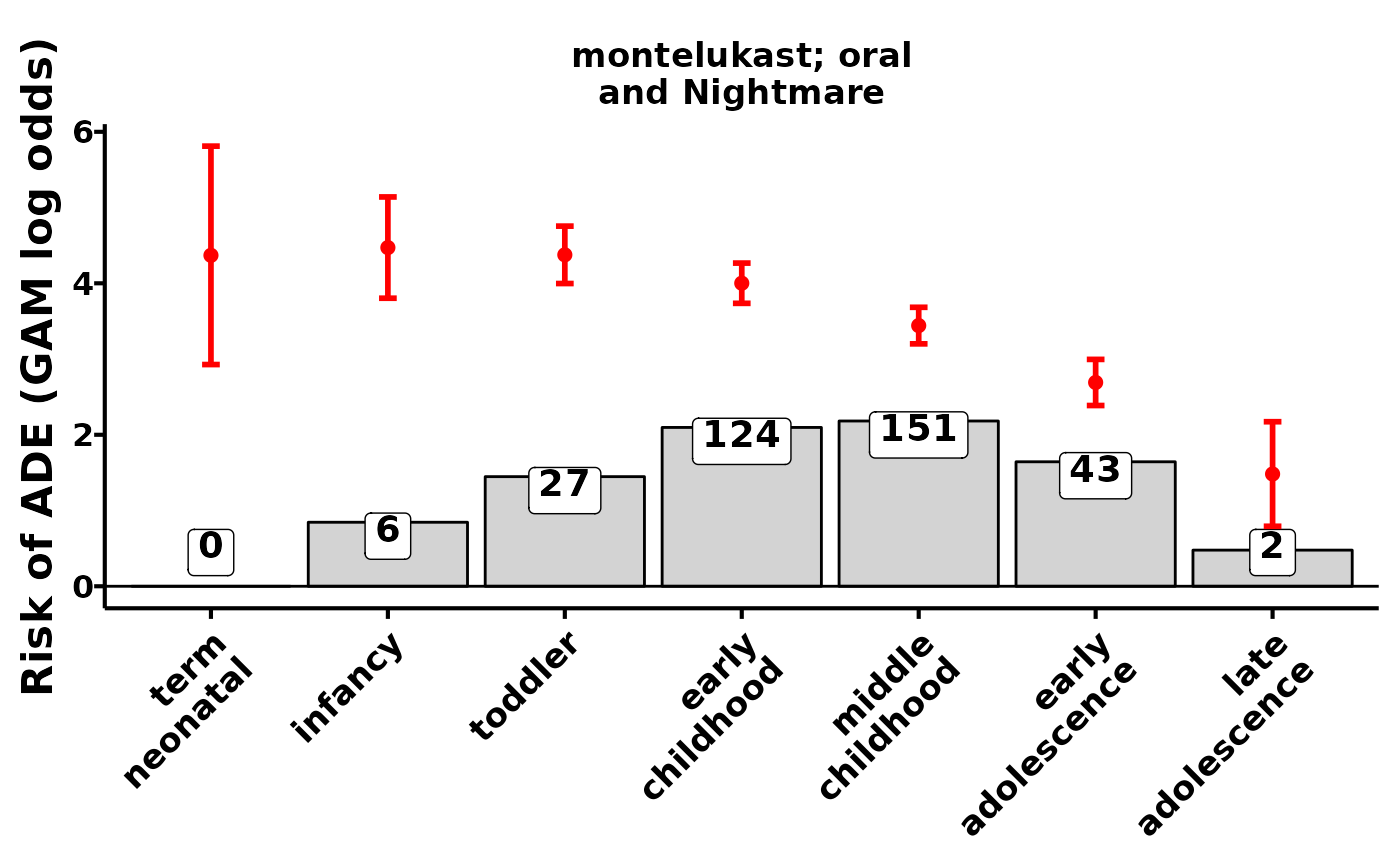## KidSIDES

The main thesis behind this published resource is that children have adverse events after drug exposure not just from the disease process but also from the biological processes from their growth and development. The National Institute of Child and Human Development defines 7 stages of child development for which we estimate signals: term neonate (0-27 days), infancy (28 days-1 years), toddler (1 year-2 years), early childhood (2-5 years), middle childhood (6-11 years), early adolescence 12-17 years, and late adolescence (18-21 years). The database contains tables of drug safety signals with drug, adverse event, and biological ontologies to facilitate teasing apart influences and contributions adverse drug events during childhood.

### An example

library(kidsides)

Connect to the database:

download_sqlite_db(force=TRUE) #downloads to cache, if not already there
#> The file will be located at at: /home/runner/.cache/R/kidsides
#>  (Yes/no/cancel)
con <- connect_sqlite_db()

Do something:

library(dplyr)
library(ggplot2)
library(tidyr)
library(stringr)

drug_ <- "montelukast; oral"
event_ <- "Nightmare"

theme_big <-
theme_classic(base_size=16) +
theme(
strip.text = element_text(color="black",face="bold"),
strip.background = element_blank(),
axis.title.x = element_blank(),
axis.line = element_blank(),
axis.ticks = element_blank(),
axis.text.x = element_text(color="black",face="bold",
angle=45,vjust=1,hjust=1),
axis.text.y = element_blank(),
axis.title.y = element_text(color="black",face="bold"),
legend.position = "top",
legend.text = element_text(color="black",face="bold"),
legend.key.size = unit(0.4,"cm"),
legend.box.margin = margin(-0.4,-0.4,0,-0.4,unit = "cm")
)

stages <-
c("term_neonatal","infancy",
"toddler","early_childhood",
stages_split <- str_replace(stages,"_","\n")

x$nichd_split <- str_replace(x$nichd,"_","\n")
x$NICHD = factor(x$nichd_split,levels=str_replace(stages,"_","\n"))
x %>%
geom_bar(stat="identity",aes(y=log10(as.numeric(DE)+1)),fill="lightgray",color="black") +
ggrepel::geom_label_repel(
aes(
label=DE,
y=log10(as.numeric(DE)+1)
),
vjust=1.3,fontface="bold",size=ts) +
geom_point(color=color,size=2) +
geom_errorbar(aes(ymin=gam_score_90mse,ymax=gam_score_90pse),
width=0.1,color=color,linewidth=1) +
geom_hline(yintercept = 0,color="black",linewidth=0.5) +
facet_wrap(~ade_name,labeller = label_wrap_gen(width = 20)) +
xlab("") +
ylab("Risk of ADE (GAM log odds)") +
theme +
theme(
axis.line = element_line(),
axis.ticks = element_line(),
axis.text.y = element_text(color="black",face="bold",size=12)
)

}

plot_ade_risks()disconnect_sqlite_db(con)